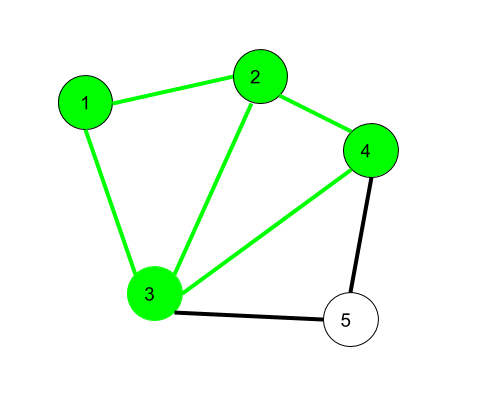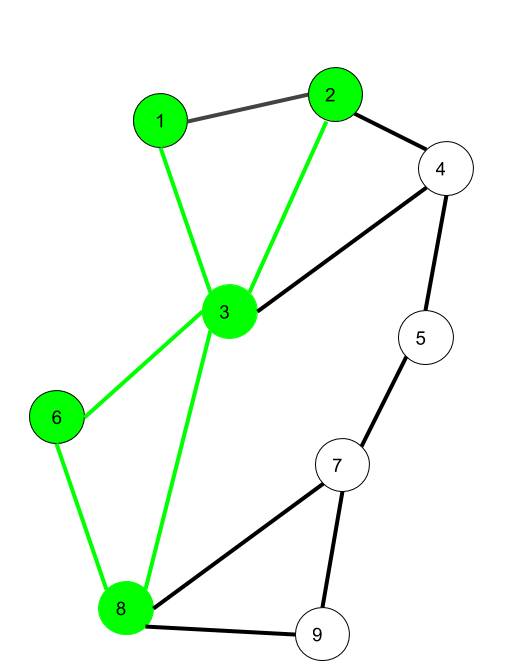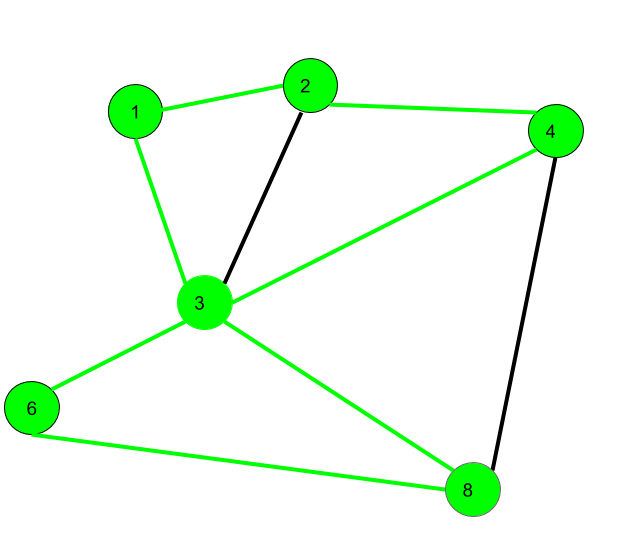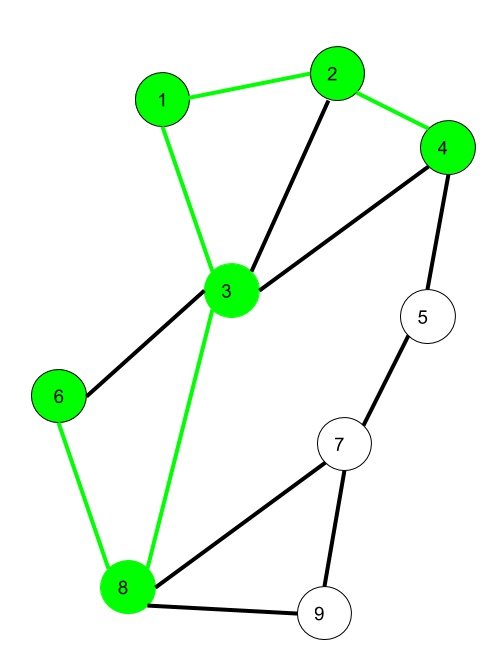# Mathematics | Walks, Trails, Paths, Cycles and Circuits in Graph

• Difficulty Level : Basic
• Last Updated : 31 Aug, 2021

Prerequisite – Graph Theory Basics – Set 1

1. Walk –
A walk is a sequence of vertices and edges of a graph i.e. if we traverse a graph then we get a walk.

Note: Vertices and Edges can be repeated.Here, 1->2->3->4->2->1->3 is a walk.

`Walk can be open or closed.`

Open walk- A walk is said to be an open walk if the starting and ending vertices are different i.e. the origin vertex and terminal vertex are different.
Closed walk- A walk is said to be a closed walk if the starting and ending vertices are identical i.e. if a walk starts and ends at the same vertex, then it is said to be a closed walk.

In the above diagram:
1->2->3->4->5->3 is an open walk.
1->2->3->4->5->3->1 is a closed walk.

2. Trail –
Trail is an open walk in which no edge is repeated.

Vertex can be repeated.Here 1->3->8->6->3->2 is trail
Also 1->3->8->6->3->2->1 will be a closed trail

3. Circuit –
Traversing a graph such that not an edge is repeated but vertex can be repeated and it is closed also i.e. it is a closed trail.

Vertex can be repeated.
Edge can not be repeated.Here 1->2->4->3->6->8->3->1 is a circuit.

```Circuit is a closed trail.
These can have repeated vertices only.```

4. Path –
It is a trail in which neither vertices nor edges are repeated i.e. if we traverse a graph such that we do not repeat a vertex and nor we repeat an edge. As path is also a trail, thus it is also an open walk.

Vertex not repeated
Edge not repeatedHere 6->8->3->1->2->4 is a Path

5. Cycle –
Traversing a graph such that we do not repeat a vertex nor we repeat a edge but the starting and ending vertex must be same i.e. we can repeat starting and ending vertex only then we get a cycle.

Vertex not repeated
Edge not repeatedHere 1->2->4->3->1 is a cycle.

```Cycle is a closed path.
These can not have repeat anything (neither edges nor vertices).```

Note that for closed sequences start and end vertices are the only ones that can repeat.

My Personal Notes arrow_drop_up
Recommended Articles
Page :